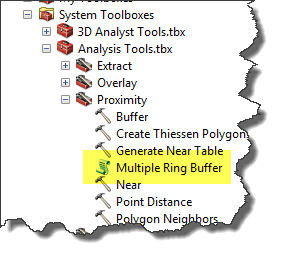# arcpy Multiple Ring Buffer analysis slow

3998
5
05-19-2016 10:01 AMRegular Contributor

I have scrip that does a multiple ring buffer and it takes 2-3 minutes to run but i use the Buffer wizard in Arcmap it takes maybe a second. Is there a different better/faster way of doing a multiple ring buffer?

There isn't much to the the arcpy multiple ring buffer.

`arcpy.MultipleRingBuffer_analysis(SP, "1_2",[1,2],"Miles","ToBufDist","ALL" )`
5 RepliesbyMVP Esteemed Contributor

If the inputs and environments are all the same, no.  The arcpy access calls the same underlying code, eventually, the delays may be in accessing the underlying code.  Direct access will always be fasterbyEsri Regular Contributor

It actually calls a script tool, it has a "script" iconbyMVP Esteemed Contributor

which means it is the fluff around the call that is slowing it downRegular Contributor

I did find that some one did try to use dictionary to store the ring buffer and create an insert cursor.

Alough my inputlayer doens't have a distance field.

```import arcpy
import string
import os
from datetime import datetime as d
startTime = d.now()

mxd = arcpy.mapping.MapDocument("CURRENT")
df = arcpy.mapping.ListDataFrames(mxd, "Layers")
lyr = arcpy.mapping.ListLayers(mxd, "SUBJECT_PROPERTY")

arcpy.env.workspace = os.path.dirname(mxd.filePath)
wp = os.path.dirname(mxd.filePath)

inputLayer = "SUBJECT_PROPERTY"
outputLayer = "1_2"

def MultiRingBuffer(ringDistance, ringCount, inputLayer, outputLayer):
buffers = []

cursor = arcpy.SearchCursor(inputLayer)
for inputFeature in cursor:
sourceOid = inputFeature.getValue("FID")
currentBuffers = dict()
buffers.append(currentBuffers)
prevBuffer = inputFeature.Shape

for multiple in range(1, ringCount + 1):
distance = multiple * ringDistance
bufferedGeom = inputFeature.Shape.buffer(distance)
bufGeom = bufferedGeom.difference(prevBuffer)
prevBuffer = bufferedGeom
row = dict()
row["FID"] = sourceOid
row["distance"] = distance
row["SHAPE"] = bufGeom
currentBuffers[distance] = row
del cursor

cursor = arcpy.InsertCursor(outputLayer)
for ringBuffers in buffers:
for feature in ringBuffers.values():
row = cursor.newRow()
for k in feature.keys():
if k == "SHAPE":
row.Shape = feature
else:
row.setValue(k, feature)
cursor.insertRow(row)
del cursor

if __name__ == '__main__':
MultiRingBuffer(1, 2, "SUBJECT_PROPERTY" ,"1_2")
print("Complete")```byEsri Esteemed Contributor

I'm getting some strange results in ArcMap 10.4, when I compare the time to buffer multi rings (1 and 2 miles) using a point featureclass with 1000 and 10000 features as a stand alone script, executing the same script in Python window and manually executing the tool:

 # features Stand alone script Python Window Toolbox 1000 40 34 22 10000 247 234 288

When using the stand alone script versus the same script (writing to different output) the standalone script will take some additional seconds to load arcpy. Using the toolbox was faster for 1000 points, but when using 10000 points the toolbox took more time than the scripts!

There are many factors that influence the process when executed in ArcMap (geoprocessing  environment settings, coordinate systems (data vs dataframe), etc).

This is the script I used for the test:

```from time import strftime
print 'start:', strftime("%Y-%m-%d %H:%M:%S")

import arcpy